Courses
Courses for Kids
Free study material
Offline Centres
More

# Rotational Motion Chapter - Physics JEE MainLast updated date: 22nd Nov 2023
Total views: 19.8k
Views today: 0.19k## Concepts of Rotational Motion for JEE Main Physics

The rotational motion of the objects plays an important role in understanding dynamics in 3D. In the past chapters, students have learned about the motion in one and two-dimensional planes, where an object will be in a straight line motion. Now the question arises will the same kinematics theory and equations be applicable if the object starts rolling? To answer this question students should study the chapter on rotational motion and understand what is rotational motion.

The rotational motion chapter deals with all the phenomena and theories related to rotation and rotational motion examples. In the rotational motion chapter, we will look at the kinematics and dynamics of a solid body in two different motions. Rotation around a stationary axis, often known as pure rotation, is the first type of motion for a solid body. The second type of solid motion is plane motion, in which the solid body's centre of mass moves in a fixed direction while the body's angular velocity remains perpendicular to that plane at all times.

Furthermore, in the rotational motion chapter, we study the rotational dynamics and the parameters associated with it. The chapter includes many important concepts such as angular velocity, angular acceleration, perpendicular axis theorem, parallel axis theorem, and torque, and we define the moment of inertia of various objects such as a ring, disc, cylinder, etc.. which are all important in exam perspective.

In this article, we will cover the concepts which are useful for the JEE exam and boost their exam preparation.

## JEE Main Physics Chapters 2024

### Important Topics of Rotational Motion Chapter

• Rigid Bodies

• Rotational Kinematics

• Moment of Inertia

• Parallel Axis Theorem

• Perpendicular Axis Theorem

• Torque

• Angular Momentum

• Angular Displacement

• Conservation of Angular Momentum

• Instantaneous Axis of Rotation

• Rolling motion

• Toppling

### Rotational Motion Important Concept for JEE Main

 Sl. No Name of Concept Key Points to Remember 1. Rigid Bodies A rigid body is basically a solid body in which the shape and size of the body remain unchanged under motion and interaction with other bodies. 2. Motion of a Rigid Body Rigid bodies undergo mainly three types of motion:1. Pure Rotational: The rotation of the body about a fixed axis is known as rotational motion. Pure rotational motion means that all the points at the same radius from the centre of rotation will have the same velocity.Pure rotational motion examples or rotary motion examples: Rotation of the ceiling fans2. Pure Translational: A rigid body is said to be in pure translational motion if any straight line fixed to it remains parallel to its initial orientation all the time.Ex: A bike/car moving along a straight long road3. Translational+Rotational: When a rigid body executes pure rotation around an axis while also translational motion with respect to a reference frame, it is said to be in combined translational and rotational motion.Ex: A rolling wheel 3. Rotational Kinematics Just like in linear kinematics, in rotational kinematics we study the parameters related to rotational motion. Such as angular velocity, angular displacement, angular acceleration, etc.. 4. Moment of Inertia Moment of Inertia plays an important role in the study of rotational dynamics. It is defined as:$I=\displaystyle\sum\limits_{i=1}^n {m_i}{r_i}^2$Where, ri- The perpendicular distance of ith particle of mass mi from the axis of rotationFor a continuous body moment of inertia is defined as:$I=\int {r^2} dm$ Where,dm- Mass of an infinitesimal element of the body at a perpendicular distance r from the axis of rotationNote: Moment of inertia does not depend on the mass of the body 5. Centre of the Mass of a Rigid Body Centre of the mass of a rigid body is an imaginary point where the mass of the entire system is concentrated with external forces focusing on this balancing point. 6. Parallel Axis Theorem Statement: The Moment of Inertia of a body about any axis is equal to the sum of the Moment of Inertia of the body about a parallel axis passing through its centre of mass and the product of its mass and the square of the distance between the two parallel axes.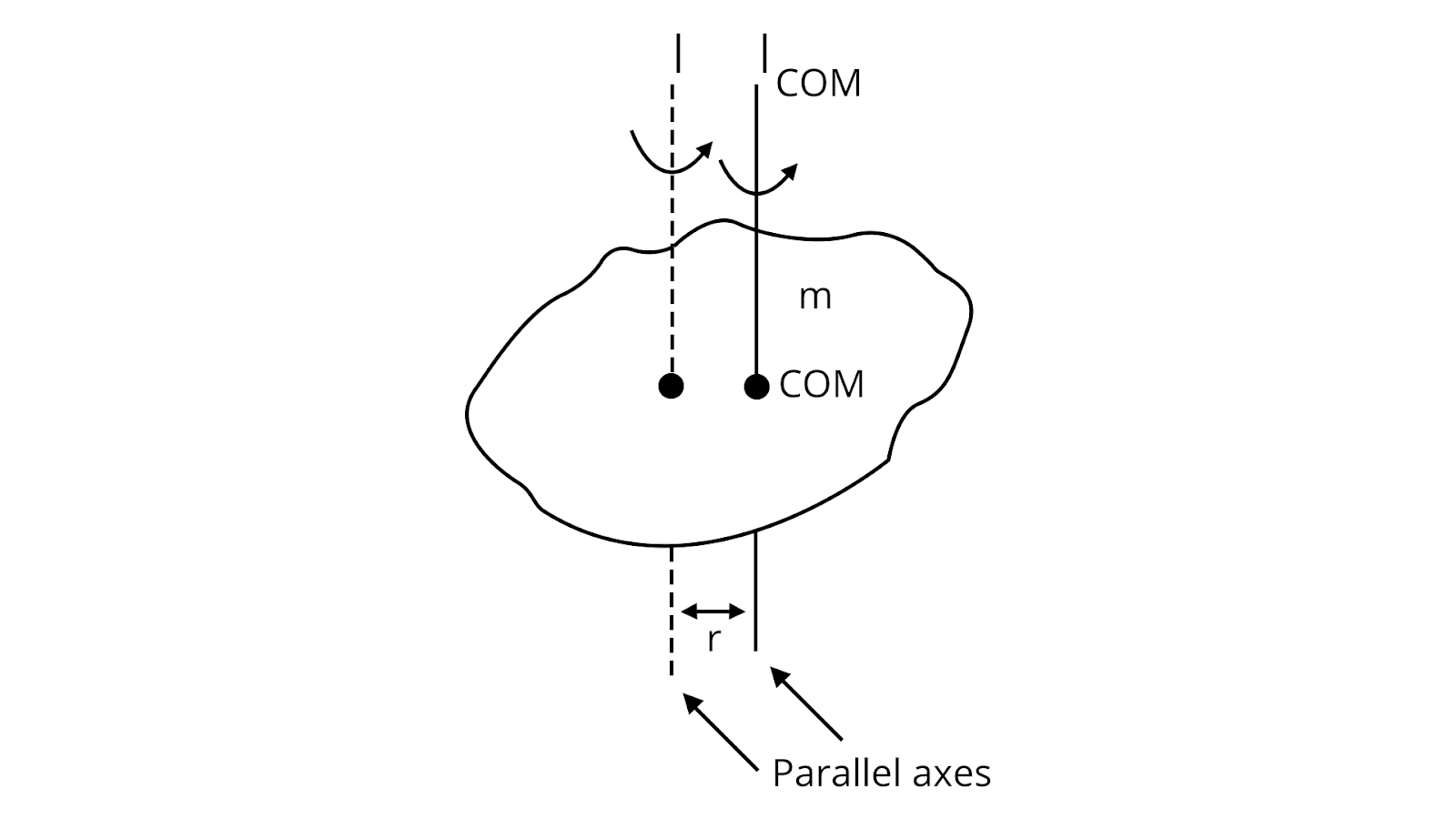$I={I_C}+M {d^2}$Where,d- The perpendicular distance between the two axes. 7. Perpendicular Axis Theorem Statement:The moment of Inertia of a planar body about an axis perpendicular to its plane is equal to the sum of its moments of inertia about two perpendicular axes concurrent with a perpendicular axis and lying in the plane of the body.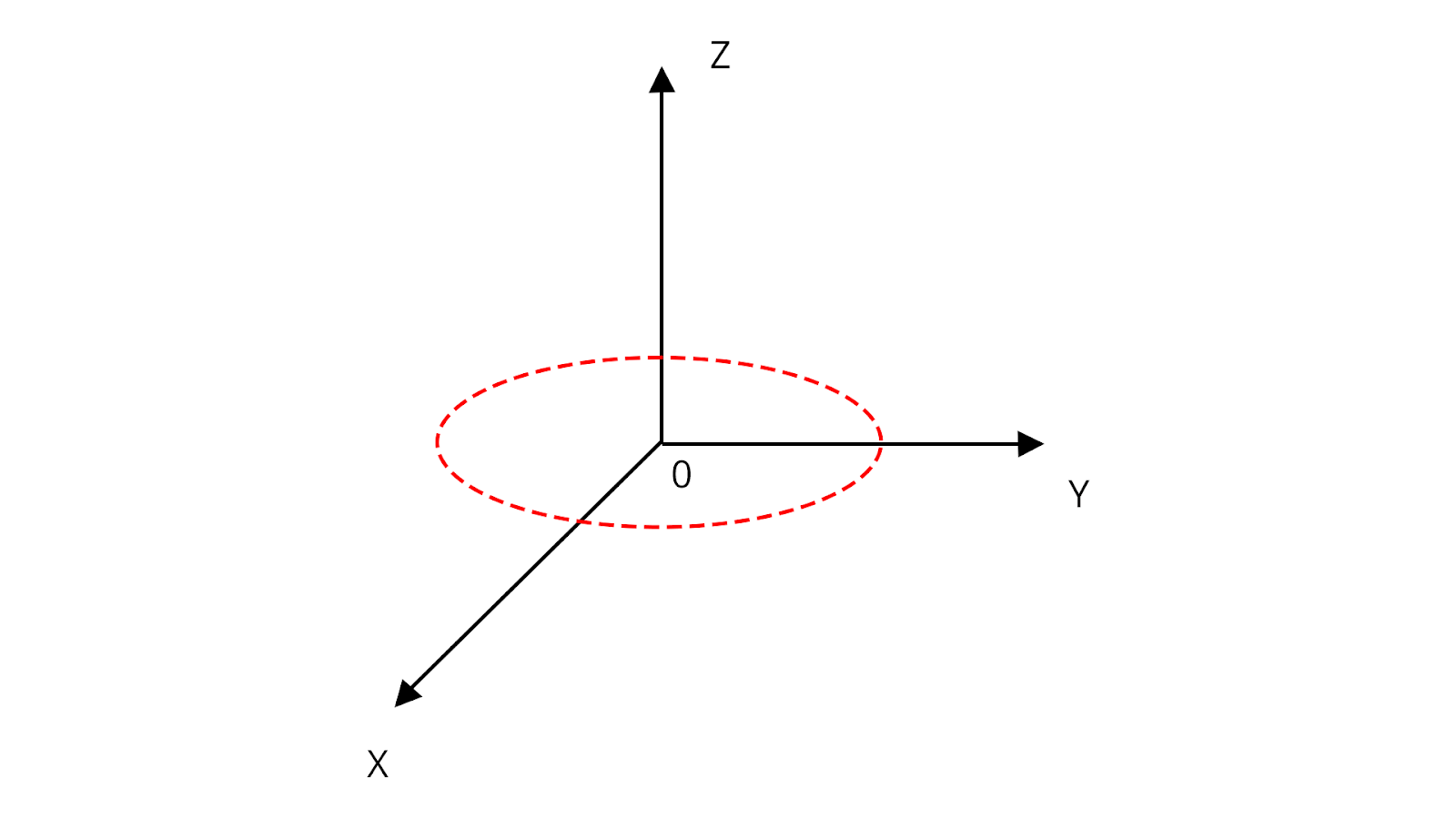${I_Z}={I_X}+{I_Y}$ 8. Angular Momentum Angular momentum is the rotational analog of linear momentum. It is a vector quantity that describes the amount of rotation of an object. 9. Angular Displacement When a rigid body rotates about a fixed axis, the angle displaced by a line passing through a point on that body and intersecting the axis of rotation perpendicularly is the angular displacement.It can be counterclockwise (positive) or clockwise (negative).It is analogous to a component of the displacement vector.Its SI unit is the radian. Other units include degree and revolution. 10. Conservation of Angular Momentum Statement:When the resultant torque acting on a system of particles is zero, then the total angular momentum vector of the system remains constant.I.e., $\vec\tau_{net}=0$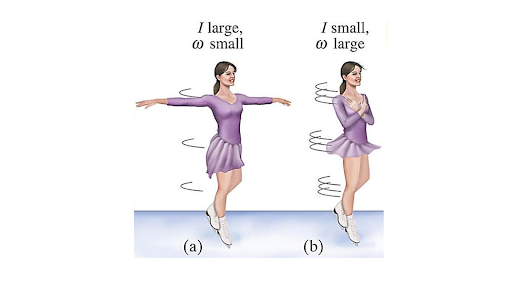11. Instantaneous Axis of Rotation The instantaneous axis of rotation (IAOR) of a rigid body is the axis fixed to the body about which the body appears to be rotating at a particular instant of time. It is also known as the instantaneous center of rotation (ICOR).To locate the IAOR of a rigid body, we can use the following methods:Method 1: Draw perpendicular lines from the velocity vectors of two points on the body. The point where the two lines intersect is the IAOR.Method 2: If the body is in pure rolling, the IAOR is the point of contact of the body with the surface on which it is rolling. 12. Rolling motion Rolling motion is a combination of translational and rotational motion. It is a type of motion in which a rigid body moves in such a way that one point on the body remains in contact with a surface at all times. This point is called the point of contact. 13. Toppling Toppling is a phenomenon in which a rigid body rotates about a fixed point until it falls over. It is a common occurrence in everyday life, such as when a child stacks blocks or when a person leans too far back in their chair. 14. Angular Velocity It is the average angular velocity and can be negative or positive.It is a vector quantity and the direction is perpendicular to the plane of rotation.The angular velocity of a particle varies at different points.The angular velocity of all the particles of a rigid body is the same as a point.It represents the speed (how fast) of an object rotating or revolving relative to another point or the speed at which the angular position or orientation of a body changes with time. 15 Torque “Torque is a unit of measurement for the force that can cause an item to revolve about an axis”. Torque is what causes an angular acceleration.Formula: $\tau =F\times r$ $\tau =F\times r\times \sin \theta$The SI unit of torque is the Newton-metre. Conventionally, the right hand grip rule is used to determine the torque vector's direction. The direction of the resulting vector is shown by the thumb if we point our index finger along vector A and middle finger along vector B 16 Types of Torque Torque can either be static or dynamic.Static Torque: Any torque that does not result in an angular acceleration is static. For example, someone pushing on a closed door is applying a static torque to the door because the door is not rotating about its hinges, despite the force applied.Dynamic Torque: A racing car's drive shaft transfers the dynamic torque because it must cause the wheels' angular acceleration if the vehicle is to accelerate along the track. 17 Rotational Equilibrium A body is in translational equilibrium if the sum of all external forces acting on it is zero. This means that the body is not accelerating, and it may be either at rest or moving with constant velocity.A body is in rotational equilibrium if the sum of all external torques acting on it is zero. This means that the body is not angularly accelerating, and it may be either at rest or rotating with constant angular velocity.If a body is in translational equilibrium and rotational equilibrium, then it is in static equilibrium. This means that the body is not accelerating in either direction.

## Torque on a Rotating Rigid Object

Some coupled forces on a rigid body cause it to rotate along its axis. These forces are called torque or moment of force. The figure below depicts the torque on a rigid body.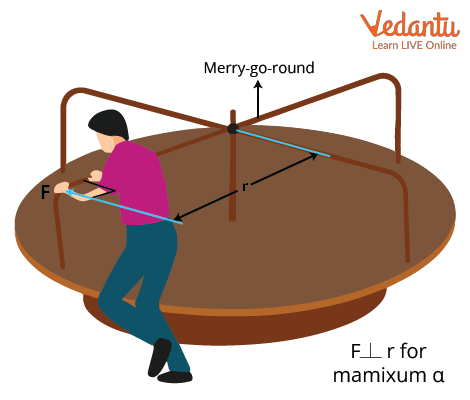## Torque on a Merry-go-round

The torque of a rotating rigid body is denoted as $\tau=r . f=r F \sin \theta$

Where, $\tau$ is the torque, F is the force applied, r is the distance between an applied force from its axis of rotation and $\theta$ is the angle between r and F.

In case the direction of F and r are parallel to one another the angle between them is zero, thus making the value of $\sin \theta$ as zero. It makes the net torque acting on a body to be equal to zero.

## Work Done in Rotational Motion

According to the work-energy principle, the total work done on a body is the sum of all the forces acting on it which is equal to the change in kinetic energy of the body.

As we know that the work done on an object is equal to the change in its kinetic energy thus work is denoted as

$W=\Delta K E=K E_{2}-K E_{1}=\dfrac{1}{2} I\left(\omega_{2-}^{2} \omega_{1}^{2}\right)$

## Power in Rotational Motion

Power for both linear and rotational motion is important. The power for a linear motion is denoted as $P=\vec{F}$ where the force is constant. In the section below the torque is considered to be constant. From the work and kinetic energy that has been derived above the power in rotational motion is defined as the rate of doing work.

$P=\dfrac{dW}{dt}$

If the net torque is constant, then work done become $W=\tau\theta$, the power then is calculated as $P=\dfrac{dW}{dt}$

On substituting the value of W we get,

\begin{align} &\mathrm{P}=\dfrac{d(\tau \theta)}{d t}\\ &\mathrm{P} =\tau \dfrac{d \theta}{d t}\\ &\text { or } P=\tau \omega \end{align}

## Rotational Dynamics

Rotational Dynamics are completely analogous to linear motion. Depicted below is the comparison between the quantities in translation or linear and rotational dynamics.

 Sr.No Quantity Linear Motion Rotational Motion 1 Force F =ma $\tau=r\cdot f=r F \sin \theta$ 2 Acceleration $a=\dfrac{d v}{d t}$ $\alpha=\dfrac{d \omega}{d t}$ 3 Velocity $v=\dfrac{d r}{d t}$ $\omega=\dfrac{d \theta}{d t}$ 4 Work $W=\int F \cdot d r$ $W=\int \tau \cdot d\theta$ 5 Power P=Fv $P=\tau \omega$ 6 Mass M I (Moment of Inertia) 7 Linear Momentum p=Mv $L=I \omega$ 8 Kinetic Energy $\dfrac{1}{2}Mv^2$ $\dfrac{1}{2}l\omega^2$ 9 Displacement dr dθ

From the table above it is clear that force in a linear motion is the torque for the rotational motion.

## List of Important Formulas for Rotational Motion Chapter

 Sl. No. Name of Concept Formula 1. Moment of Inertia of circular-shaped bodies Ring: $I= M {R^2}$Disc : $I=\dfrac { M{R^2}}{2}$Solid sphere: $I=\dfrac {2}{5} M {R^2}$Spherical shell: $I=\dfrac {2}{3} M{R^2}$ 2. Moment of inertia of cylindrical bodies Hallow cylinder: $I= M {R^2}$Solid cylinder: $I=\dfrac { M{R^2}}{2}$Thick-walled cylinder: $I=\dfrac {M \left ({R_1}^2+{R_2}^2\right )}{2}$Solid cylinder of length l: $I=\dfrac {M{R^2}}{4} +\dfrac {M {l^2}}{12}$ 3. Moment of inertia of a thin rod Thin rod of length l: $I=\dfrac {M{l^2}}{12}$Special case:If axis of rotation is Perpendicular to rod at one end.$I=\dfrac {M{l^2}}{3}$ 4. Moment of inertia of a rectangular sheet $I=\dfrac {M \left ({l^2}+{b^2}\right )}{2}$ 5. Torque $\tau =\vec r \times \vec F$ 6. Radius of gyration $K=\sqrt {\dfrac {I}{M}}$ 7. Rotational work and energy Rotational work: $W_{rot}=\int \tau d\theta$Kinetic energy: $k_{rot}=\dfrac {1}{2} I {\omega^2}$ 8. Angular momentum $L=\vec r \times \vec P$ 9. Angular momentum of a rigid body in combined translational and rotational motion $\vec L=\vec {L_{cm}}+M\left ( \vec {r_o}+\vec {v_o}\right )$ 10. Angular impulse $\vec J=\Delta \vec L$

## Important Comparison of Rotational Kinematics and Linear Kinematics

When we start preparing for our exams its essential to know our understanding with the help of comparisions, differences between the concepts we have previously studied. We know the kinematics of linear motion, now let us have a look at the comparison of linear kinematics with rotational motion.

 Rotational Kinematics Linear Kinematics Linear position is denoted by S Angular position is denoted by $\theta$ Displacement: $\Delta S= {S_2}- {S_1}$ Angular Displacement: $\Delta {\theta}={\theta_2}-{\theta_1}$ Average velocity: $v_{avg}=\dfrac {\Delta S}{\Delta t}$ Angular average velocity: $\omega_{avg}=\dfrac {\Delta \theta}{\Delta t}$ Instantaneous velocity: $v_{ins}= \lim_{{\Delta t} \rightarrow 0} \dfrac {\Delta S}{\Delta t}$ Instantaneous angular velocity: ${\omega_{ins}}=\lim_{{\Delta t} \rightarrow 0} \dfrac {\Delta \theta}{\Delta t}$ Average acceleration: $a_{avg}=\dfrac {\Delta v}{\Delta t}$ Average angular acceleration: ${\alpha_{avg}}=\dfrac {\Delta \omega}{\Delta t}$ Instantaneous acceleration:$a_{ins}=\lim_{{\Delta t} \rightarrow 0} \dfrac {\Delta v}{\Delta t}$ Instantaneous angular acceleration:${\alpha_{ins}}=\lim_{{\Delta t} \rightarrow 0} \dfrac {\Delta \omega}{\Delta t}$ Linear equations of motion:$v={v_o}+at$${v^2}={{v_o}^2}+2as$$s={v_o}t+\dfrac {1}{2} a{t^2}$ Rotational equations of motion:$\omega = {\omega_o}+\alpha t$${\omega^2}={\omega_o}^2+2 \alpha \theta$$\theta={\omega_o}t+\dfrac {1}{2} \alpha {t^2}$

### JEE Main Rotational Motion Solved Examples

1. The moment of inertia of a uniform cylinder of length and radius R about its perpendicular bisector is I. What is the ratio l/R such that the moment of inertia is minimum?

Sol:

Given,

We have a uniform cylinder of length l and radius R, such that the pendicular bisector is I. Now we are asked find the ratio $\dfrac {l}{R}$.

We know that, moment of inertia of a cylinder of length l is:

$I=\dfrac {M{R^2}}{4} +\dfrac {M {l^2}}{12}$

$I=\dfrac {M^2}{4{\rho}{\pi}l}+\dfrac {M{l^2}}{12}$

For maximum I, we have $\dfrac {dI}{dl}=0$

$\dfrac {dI}{dl}=\dfrac {-M^2}{4{\pi}{\rho}{l^2}}+\dfrac {M{l}}{6}=0$

$\dfrac {M^2}{4{\pi}{\rho}{l^2}}=\dfrac {M{l}}{6}$

$\dfrac {M}{{4{\pi}{\rho}}}=\dfrac {l^3}{6}$

$\dfrac {4 \pi \rho {R^2}{l}}{{4{\pi}{\rho}}}=\dfrac {l^3}{6}$

$\dfrac {l^2}{R^2}=\dfrac {3}{2}$

$\dfrac {l}{R}=\sqrt {\dfrac {3}{2}}$

Therefore, the ratio of $\dfrac {l}{R}$ is $\sqrt {\dfrac {3}{2}}$.

Key point: Here we should use the formula of volume mass density $\rho=\dfrac {m}{4\pi {R^2} l}$. While solving problems related to cylinder or any other geometrical objects we must remember that the mass density will vary from object to object.

1. Seven identical circular planar disks, each of mass M and radius R are welded symmetrically as shown. The moment of inertia of the arrangement about the axis normal to the plane and passing through the point P is?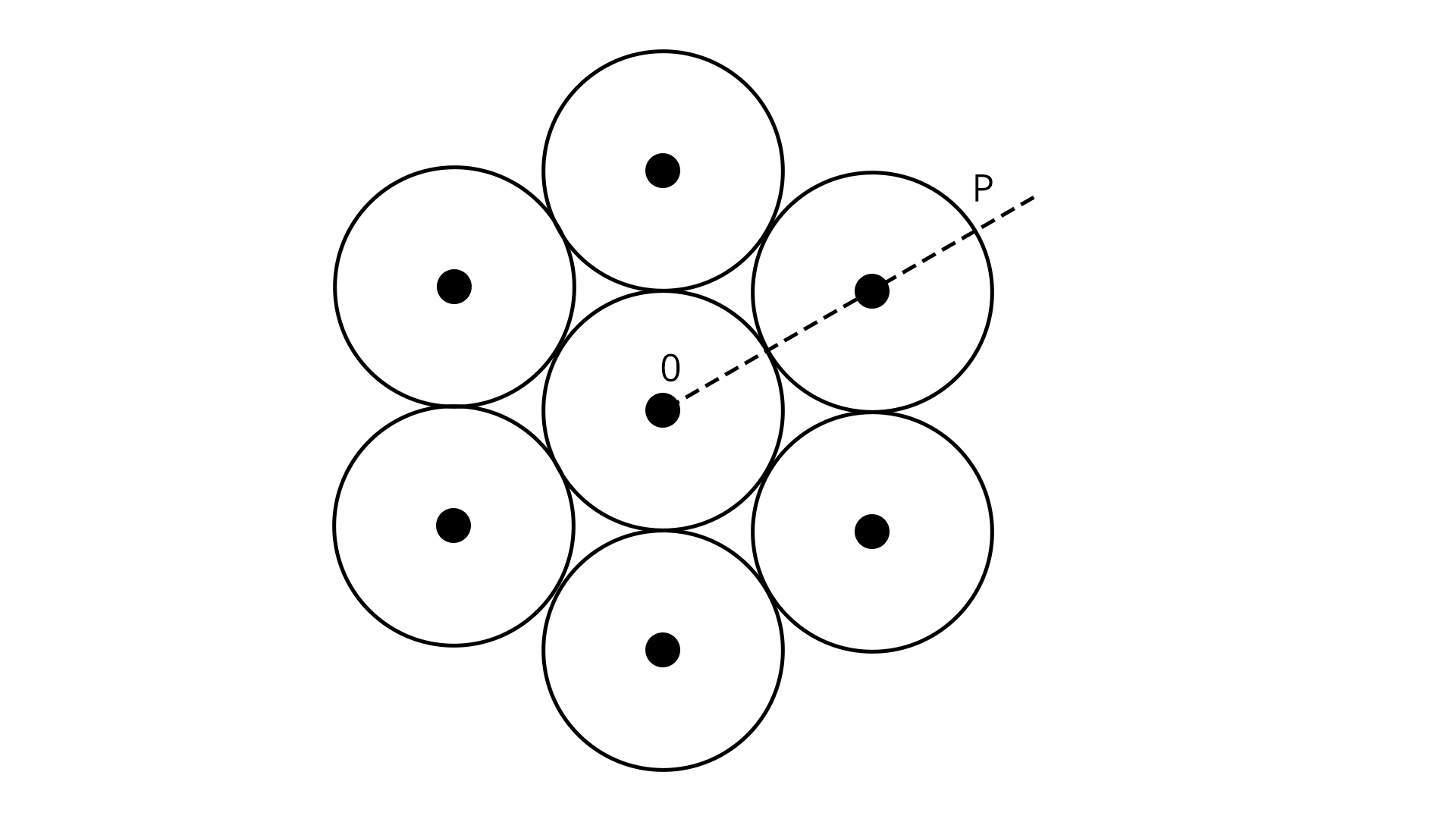Sol:

Here, the arrangement of seven identical disks are welded symmetrically, thus all the discs will rotate about center of mass. We know that, according to the parallel axis theorem, The moment of inertia of a body about any axis is equal to the sum of the Moment of Inertia of the body about a parallel axis passing through its centre of mass and the product of its mass and the square of the distance between the two parallel axes.

I.e.,

$I={I_C}+M {d^2}$

Where,

d- The perpendicular distance between the two axes.

${I_o}=\dfrac {7M{R^2}}{2}+6M {{\left (2R\right)}^2}=\dfrac {55 M{R^2}}{2}$

Now,  The moment of inertia of the arrangement about the axis normal to the plane and passing through the point P is:

${I_P}={I_o}+M{d^2}$

${I_P}=\dfrac {55 M{R^2}}{2}+7M{\left (3R\right )}^2=\dfrac {181}{2} M{R^2}$

Key point: While solving this question, we must first calculate the moment of inertia of the entire system of seven particles, and then we can calculate the moment of inertia at any point on the disk with the help of parallel axis theorem.

### Previous Year Questions of Rotational Motion

1. A 2 kg steel rod of length 0.6 m is clamped on a table vertically at its lower end and is free to rotate in the vertical plane. The upper end is pushed so that the rod falls under gravity, ignoring the friction due to clamping at its lower end, the speed of the free end of the rod when it passes through its lowest position is ____________ ms-1. (Take g = 10 ms-2) (JEE 2021)

Sol:

Given,

Mass of the steel rod=M= 2 kg

Length of the steel rod=l=0.6 m

The given rod is clamped on a table vertically at its lower end and its free to rotate in a vertical plane. Now, we are asked to determine the speed of the free end of the rod when it passes through its lowest position.

Here, we can use the conservation of energy to obtain the speed. From conservation of energy we get:

P.E=K.E

$mgl=\dfrac {1}{2} I {\omega^2}$

$mgl=\dfrac {1}{2} \dfrac {m {l^2}{\omega^2}}{3}$

$\omega =\sqrt {\dfrac {6g}{l}}$

Speed $v=l\omega=l\times \sqrt {\dfrac {6g}{l}}=\sqrt {6gl}$

$v=6 m/s$

Therefore, the speed of the free end of rod when it passes through its lowest position 6 m/s.

Trick: Here, we can notice that the one end of the rod is fixed, thus we can use the moment of inertia formula when the axis of rotation is perpendicular to the rod at one end i.e., $I=\dfrac {M{l^2}}{3}$.

1. The centre of mass of the solid hemisphere of radius 8 cm is x from the centre of the flat surface. Then value of x is __________. (JEE 2020)

Sol:

We know that centre of mass of a solid hemisphere is,

$x=\dfrac {3R}{8}=\dfrac {3\times 8}{8}=3 cm$

Therefore, the value of x is 3 cm.

Trick: For this kind of problem we need to recall and practice COM of a few geometrical shapes, which will save our time calculation.

### Practice Questions

1. A uniform thin bar of mass 6 kg and length of 2.4 meters is bent to make an equilateral hexagon. The moment of inertia about an axis passing through the centre of mass and perpendicular to the plane of a hexagon is _______  10-1 kg m2

(Ans: 8)

2. A small bob tied at one end of a thin string of length 1 m is describing a vertical circle so that the maximum and minimum tension in the string is in the ratio 5: 1. The velocity of the bob at the highest position is ________ m/s. (Take g = 10 m/s2)

(Ans: 5)

3. What is the physical significance of the moment of inertia?

## JEE Main Physics Rotational Motion Study Materials

Here, you'll find a comprehensive collection of study resources for Rotational Motion designed to help you excel in your JEE Main preparation. These materials cover various topics, providing you with a range of valuable content to support your studies. Simply click on the links below to access the study materials of Rotational Motion and enhance your preparation for this challenging exam.

 JEE Main Rotational Motion Study Materials JEE Main Rotational Motion Notes JEE Main Rotational Motion Important Questions JEE Main Rotational Motion Practice Paper

## JEE Main Physics Study and Practice Materials

Explore an array of resources in the JEE Main Physics Study and Practice Materials section. Our practice materials offer a wide variety of questions, comprehensive solutions, and a realistic test experience to elevate your preparation for the JEE Main exam. These tools are indispensable for self-assessment, boosting confidence, and refining problem-solving abilities, guaranteeing your readiness for the test. Explore the links below to enrich your Physics preparation.

 JEE Main Physics Study and Practice Materials JEE  Main Physics Previous Year Question Papers JEE Main Physics Mock Test JEE Main Physics Formula JEE Main Sample Paper JEE Main Physics Difference Between

### Conclusion

In this article, we discussed one of the important chapters for JEE, rotational motion. We have covered most of the important concepts, formulae, and solved examples along with recently asked questions in JEE exams. In this article, we have also studied how linear kinematics is different from the rotational kinematics and had an overview of the rotational kinematic equations and what rotary motion, translational motion, etc... This article will help students prepare for JEE exam and boost their preparation in one stance.

## FAQs on Rotational Motion Chapter - Physics JEE Main

1. Is rotational motion important for JEE?

When it comes to the physics section of JEE advanced exams, mechanics make up a large portion of the problems, with rotational mechanics being the most crucial. Rotational motion includes the concepts that carry most of the important formulae for the calculation of JEE questions.

2. What is the weightage of rotational motion in JEE main?

The rotational motion chapter is having high importance because of its vast syllabus and concepts. The chapter plays an important role in the mechanics part with a weightage of 6% which makes 3-4 questions (2 from dynamics and 2 from centre of mass).

3. Is rotational motion hard?

Rotational Motion (Rotational Mechanics) is considered to be one of the tricky topics in Class 11 JEE Physics. With the help of continuous practice and understanding of the formulas, students can easily solve questions from the rotational motion chapter.

### Repeaters Course for JEE 2022 - 23## Notice board

JEE News
JEE Blogs